很多人看到标题可能会疑惑为什么要写这个路由器的登录过程？一般来说路由器的登录不都是

我自己没有这个路由器，找朋友借的路由器，借来后上电连LAN，开IE用HttpWatch开始抓包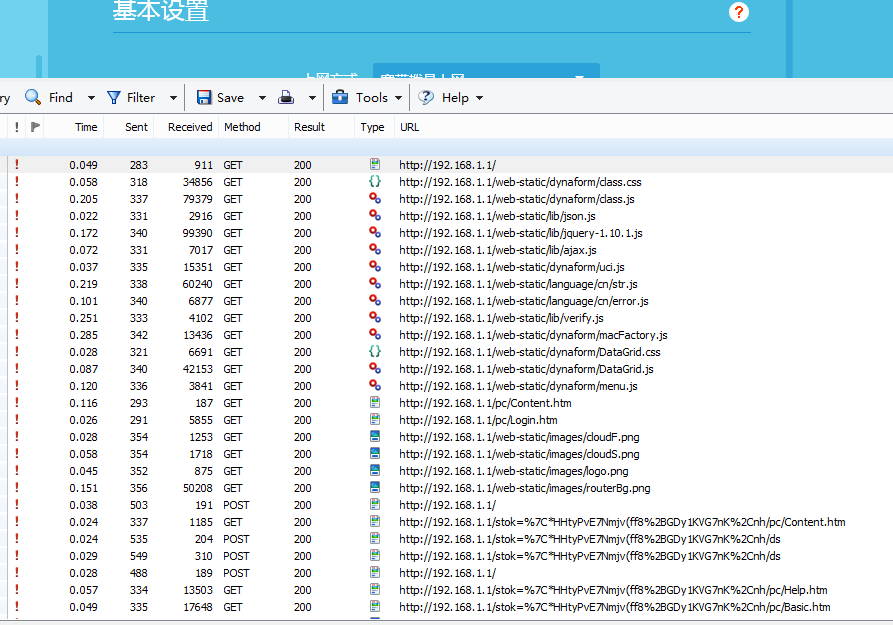﻿<!DOCTYPE html>
<html xmlns="http://www.w3.org/1999/xhtml">
<title>TL-WR886N</title>
<meta name="MobileOptimized" content="240">
<meta name="viewport" content="width=device-width, height=device-height, initial-scale=1.0, minimum-scale=0.5, maximum-scale=2.0, user-scalable=yes">
<link rel="Shortcut Icon" href="../web-static/images/icon.png" type="image/jpeg" />
<script type="text/javascript" src="../web-static/dynaform/class.js"></script>
<body>
<div id="Error"></div>
<div id="Confirm"></div>
<div id="Con"></div>
<div id="Help"></div>
<div id="Cover"></div>
<script type="text/javascript">
var gBeInCNA = "NO";
</script>
</body>
</html>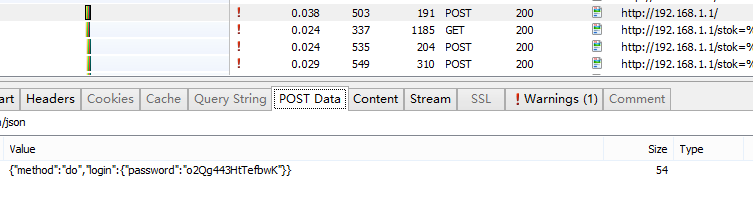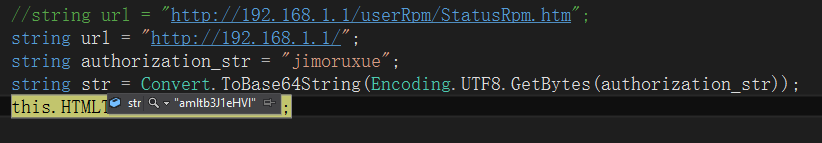Oh, Shit。竟然不是Base64加密，那会是怎么加密的？那就往上找看有没有加密的函数，上面有好几个JS，还有两个

htm文档，先看看Content.htm，里面就一个JSON对象，

{"error_code":-40401, "data":{"code":-40407,"time":20,"group":0}}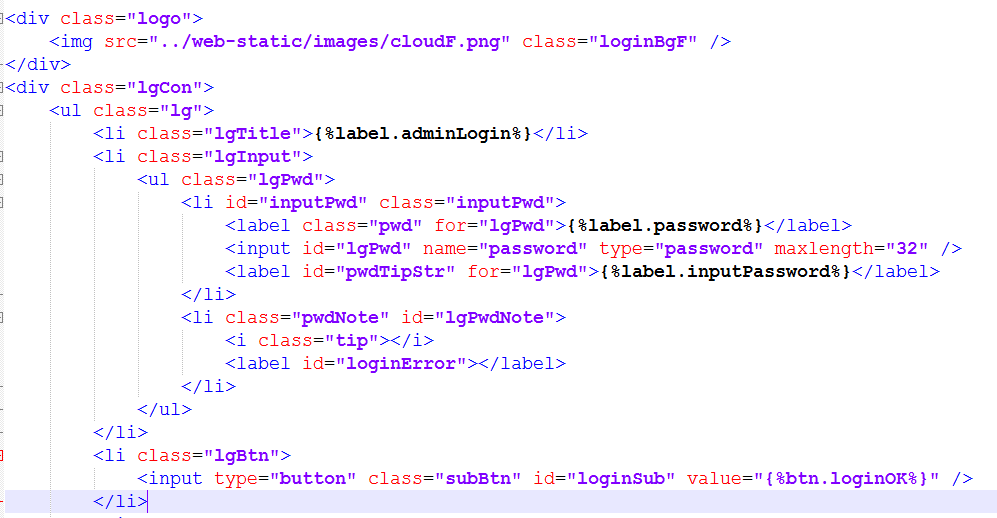没办法了，并且已经到了饭点，于是先去吃饭，回来再看看有没有其它办法来获取加密算法。吃完饭后又到网上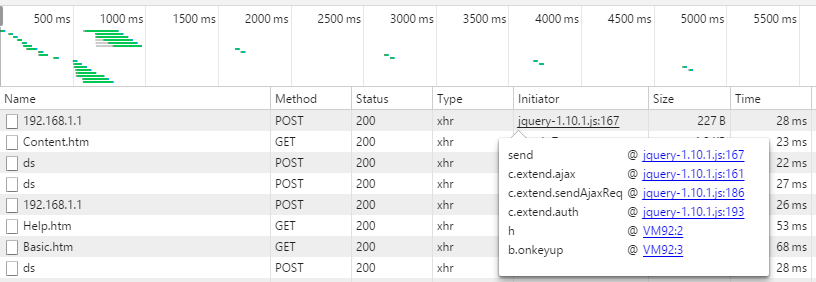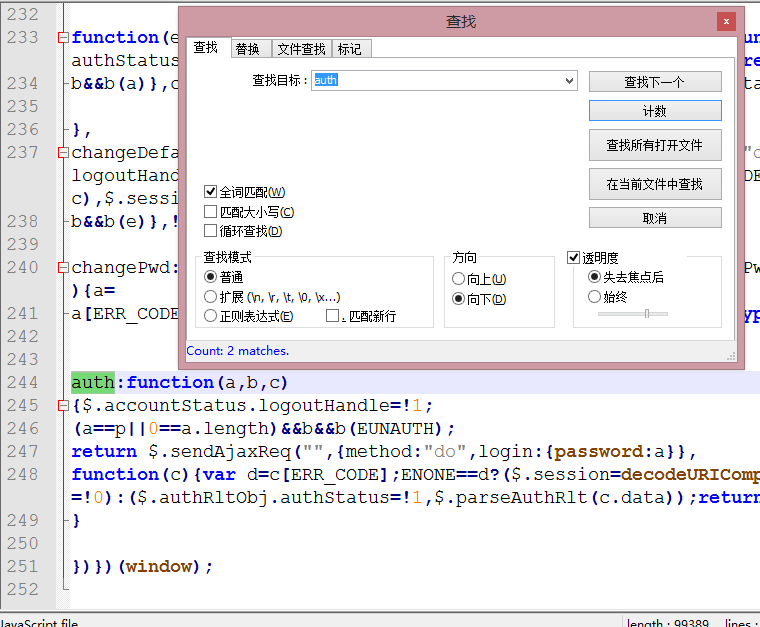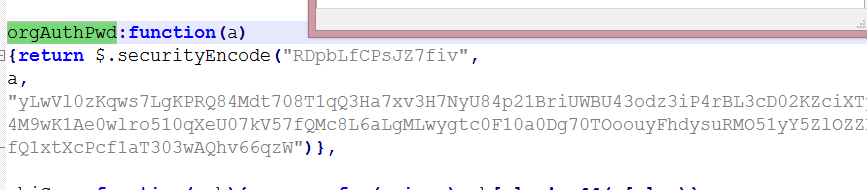securityEncode:function(a,b,c)
{
var e="",f,g,h,k,l=187,n=187;
g=a.length;
h=b.length;
k=c.length;
f=g>h?g:h;
for(var p=0;p<f;p++)
n=l=187,
p>=g?n=b.charCodeAt(p):p>=h?l=a.charCodeAt(p):(l=a.charCodeAt(p),n=b.charCodeAt(p)),e+=c.charAt((l^n)%k);return e},

//a:短验证码
//b:密码
//c:长验证码
public string securityEncode(string a,string b,string c)
{
string e="";
int f,g,h,k,l=187,n=187;
g=a.Length;//短验证码的长度(固定为15)
h=b.Length;//密码长度
k=c.Length;//长验证码的长度(固定为255)
if(g>h)//将短验证码字符串和密码字符串的长度进行比较
{
f = g;
}
else
{
f = h;
}
//f取长的那个字符串的长度
for(int p=0;p<f;p++)
{
n=l=187;
if(p>=g)
{
n=b.Substring(p, 1).ToCharArray();//n取密码中的以0开始的第p位字符
}
else
{
if(p>=h)
{
l=a.Substring(p, 1).ToCharArray();//l取短验证码中的以0开始的第p位字符
}
else
{
l=a.Substring(p, 1).ToCharArray();//l取短验证码中的以0开始的第p位字符
n=b.Substring(p, 1).ToCharArray();//n取密码中的以0开始的第p位字符
}
}
//每次计算出l和n的值之后对其取异或然后除以k(也就是除以255),
//取长验证码中以0开始的第(l^n)%k位，然后拼接到字符串e的后面
e+=c.Substring((l^n)%k,1);
}

return e;//返回加密后的密码
}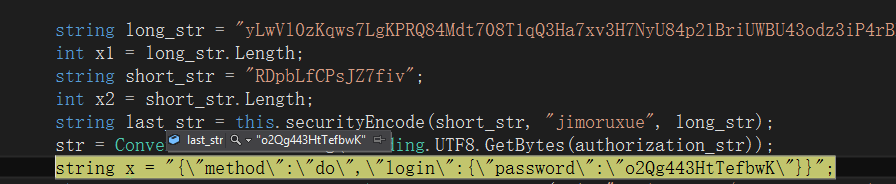HttpWebRequet的GetRequestStream方法一直报错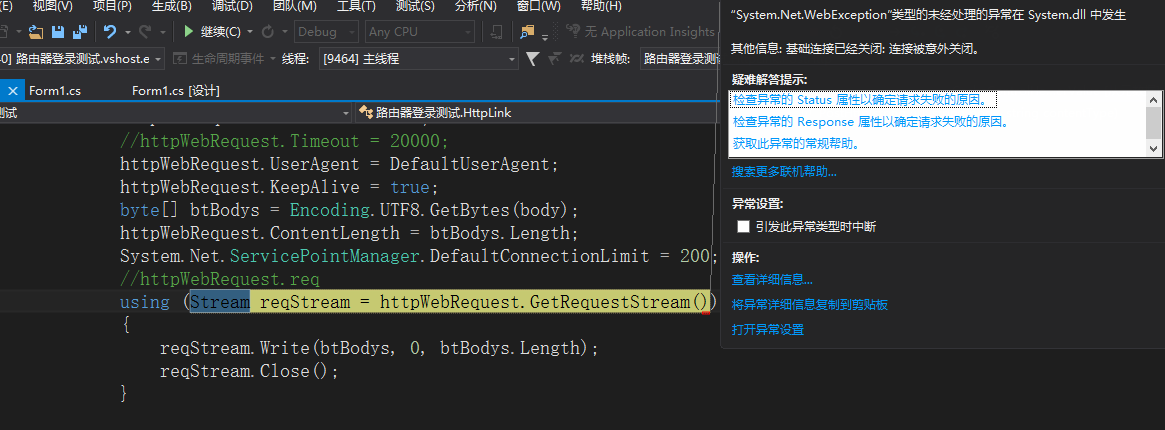©️2019 CSDN 皮肤主题: 大白 设计师: CSDN官方博客